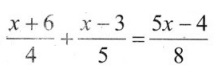# Grade 8 Linear Equations in One Variable Worksheets

## Grade 8 Maths Linear Equation in One Variable Multiple Choice Questions (MCQs)

1. The solution of 2x – 3 = 7 is:
(a) 2
(b) -2
(c) 5
(d) -5

2. Which of the following is not a linear equation.
(a) 2x + 5 = 1
(b) x – 1 = 0
(c) y + 1 = 0
(d) 5x + 3

3. The present age of Sahil’s mother is three times the present age of Sahil. After 5 years their ages will add to 66 years. Find the present age of Sahil.
(a) 12
(b) 14
(c) 16
(d) 20

4. Find the solution of 2x + 3 = 7
(a) 2
(b) -2
(c) 3
(d) None of these

5. Solve: 8x = 20 + 3x
(a) 4
(b) -4
(c) 2
(d) None of these

6. Solve:$\frac{2}{3} x+1=\frac{7}{3}$
(a) 2
(b) -2
(c) 3
(d) None of these

7. Solve:$\frac{x}{4}+\frac{x}{6}=x-7$
(a) 12
(b) -12
(c) 3
(d) None of these

8. Find the solution of$\frac{3 x+5}{2 x+1}=\frac{1}{3}$
(a) 2
(b) -2
(c) 3
(d) None of these

9. Find the solution of(a) 8
(b) -8
(c) 4
(d) None of these

10. Solve: 8x + 3 = 27
(a) 3
(b) -3
(c) 2
(d) None of these

11. Solve : 5x – 7 = 2x + 8
(a) 5
(b) -9
(c) 5
(d) 9

12. The perimeter of a rectangle is 13 cm and its width is 2$\frac{3}{4}$ cm. Find its length in cm
(a) 3$\frac{3}{4}$
(b) -3$\frac{3}{4}$
(c) 2$\frac{3}{4}$
(d) None of these

13. Two numbers are in the ratio 5 : 3. If they differ by 18, what are the numbers?
(a) 45, 27
(b) 50, 32
(c) 40, 22
(d) None of these

14. Solve: 2x -3 = x + 2
(a) 5
(b) -9
(c) 5
(d) 9

15. Solve: 3x = 2x + 18
(a) 18
(b) -18
(c) 14
(d) None of these

16. Solve: 5t – 3 = 3t – 5
(a) 1
(b) -1
(c) 2
(d) None of these

17. Solve: 5x + 9 = 5 + 3x
(a) 2
(b) -2
(c) 3
(d) None of these

18. Solve: 4z + 3 = 6 + 2z
(a)$\frac{3}{2}$
(b) –$\frac{3}{2}$
(c) 2
(d) None of these

19. Solve: 2x – 1 = 14 – x
(a) 5
(b) -9
(c) 5
(d) 9

20. Solve: 8x + 4 = 3(x – 1) + 7
(a) 1
(b) -1
(c) 0
(d) None of these

### Class 8 Maths Linear Equation in One Variable Solve

1. Solve: 2y + 9 = 4
2. Solve:$\frac{x}{3}+\frac{5}{2}=\frac{-3}{2}$
3. Solve:$\frac{15}{4}$ – 7x = 9
4. Solve: x – 2 = 7
5. Solve: y + 3 = 10
6. Solve: 6 = z + 2
7. Solve: 6x = 12
8. Solve:$\frac{x}{5}$ = 10
9. Solve:$\frac{2x}{3}$ = 18
10. Solve: 7x – 9 = 12

### Class 8 Maths Linear Equation in One Variable Short Answer Type Questions

1. Interior angles of a triangle are in the ratio 2 : 3 : 4. Find the angles of the triangle.
2. If father is twice as old as his son and also 32 years older than his son. What is the age of father?
Solve for: 8x + 25 = 4x +105
4. Sides of rectangle are is the ratio 15 : 4. If the perimeter of the rectangle is 38 cms then find the sides of rectangle.
5. Form an equation of “7 added to thrice a number is 118” and also find the number.
6. If sum of two numbers is 29 and one of them is 18. Form the equation for finding the another number.

### Class 8 Maths Linear Equation in One Variable Long Answer Type Questions

1. A number consists of two digits whose sum is 9. If 9 is added to the number its digits are inter changed. Find the number.
2. The sum of the two number is 5 and difference of their squares is 5. Find the difference of the numbers?
3. The sum of four consecutive natural numbers is 166. What are the numbers?
4. A man ₹ x. He gave half of it to his wife,$\frac{1}{4}^{t h}$ to his son and ₹ 1200 of his daughter. Form an equation and also find x.
5. For what value of y is the perimeter of shape 220 cm?